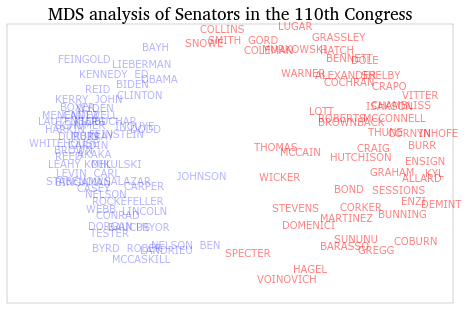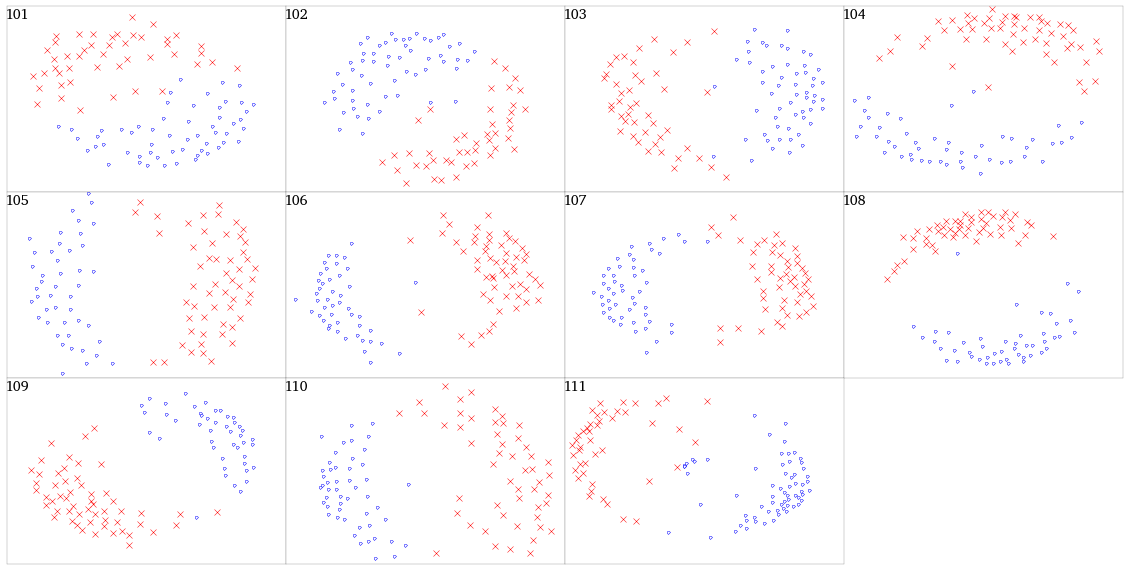In :
%pylab inline

Welcome to pylab, a matplotlib-based Python environment [backend: module://IPython.zmq.pylab.backend_inline].

In :
import numpy as np
import matplotlib.pyplot as plt
from pandas import *
from sklearn.manifold import MDS
from statsmodels.iolib.foreign import genfromdta
import os
from sklearn.metrics.pairwise import euclidean_distances
import re
import math


# Distance metrics of Senators by voting record¶

The data live in the data/roll_call subfolder. These were converted to CSV using R's read.dta function in the foreign package.

Converting them with statsmodels failed, presumably due to an incompatible Stata version (statsmodels can only read data from certain versions of Stata.)

In :
datdir = os.path.abspath(os.path.join('data', 'roll_call'))
csv_files = [os.path.join(datdir, f) for f in os.listdir(datdir)
if f.endswith('.csv')]


We'll store the date for each Congress in a DataFrame, and collect all of them in a dictionary. We can then get the data for the 110th Congress, for example, by calling roll_call.

In :
roll_call = {}
for f in csv_files:
s = re.search('sen(.*)kh', f)
congnum = int(f[(s.start()+3):(s.end()-2)])


Transform the voting codes to a simplified Yea (1), Nay (-1), No-vote (0) scheme.

In :
def roll_call_simplified(df):
vote_codes = {1:  1,
2:  1,
3:  1,
4: -1,
5: -1,
6: -1,
7:  0,
8:  0,
9:  0,
0:  0}

# Remove the vice-president.
no_pres = df[df.state < 99]

# Find the columns with vote data. These are typically 'V1', 'V915', etc.,
# but some dataset appear to have them as 'var1', 'var919', etc.
# Checking that the first letter is 'v' or 'V' will find these (and only)
# these columns.
vote_cols = [c for c in df.columns if c.upper() == 'V']

# Would like to just call replace on the whole df, but doesn't seem
# to work, so we'll apply replace to each column.
no_pres[vote_cols] = no_pres[vote_cols].apply(lambda x: x.replace(vote_codes))
return no_pres

In :
for cong in roll_call:
roll_call[cong] = roll_call_simplified(roll_call[cong])


We use scikit-learn's euclidean_distances function to compute the euclidean distances between observations (senators) in each Congress. The function takes a matrix of shape (No. of obs.) $\times$ (No. of features/variables).

In :
distance = {}
for cong in roll_call:
vote_cols = [c for c in roll_call[cong].columns if c.upper() == 'V' ]
distance[cong] = euclidean_distances(roll_call[cong][vote_cols].values)


Compute the MDS coordinates for the 110th Congress, and plot them.

In :
rc110 = roll_call
dist110 = distance
dems = np.where(rc110.party == 100)
repubs = np.where(rc110.party == 200)
names = roll_call.name.values

mds = MDS().fit_transform(dist110)

plt.figure(figsize = (8, 5))
# Plot invisible points to annotate with names
plt.plot(mds[:, 0], mds[:, 1], '.', alpha = 0)
plt.title('MDS analysis of Senators in the 110th Congress')
# Plot dem names
for i in dems:
plt.annotate(names[i], (mds[i, 0], mds[i, 1]),
color = 'blue',
alpha = 0.3,
horizontalalignment = 'center',
verticalalignment = 'center',
family = 'sans-serif')
# Plot repub names
for i in repubs:
plt.annotate(names[i], (mds[i, 0], mds[i, 1]),
color = 'red',
alpha = 0.5,
horizontalalignment = 'center',
verticalalignment = 'center',
family = 'sans-serif')

# Turn off axes labels.
plt.setp(plt.gca().get_yaxis(), visible = False)
plt.setp(plt.gca().get_xaxis(), visible = False)

Out:
[None]And do the same for every Congress.

In :
fig, ax = plt.subplots(nrows = 3, ncols = 4, figsize = (20, 10))
plt.subplots_adjust(hspace = 0, wspace = 0)
for a, cong in zip(ax.ravel(), roll_call):
rc = roll_call[cong]
dist = distance[cong]
dems = np.where(rc.party == 100)
repubs = np.where(rc.party == 200)

mds = MDS().fit_transform(dist)

a.plot(mds[dems, 0], mds[dems, 1], '.b', mfc = 'white')
a.plot(mds[repubs, 0], mds[repubs, 1], 'xr')
# Label which Congress is being plotted
a.text(0, .99, cong, transform = a.transAxes,
verticalalignment = 'top', fontsize = 14)
# Turn off ticklabels
plt.setp(a.get_yaxis().get_ticklabels(), visible = False)
plt.setp(a.get_xaxis().get_ticklabels(), visible = False)

# Turn off the 12th subplot
plt.setp(ax[2, 3], visible = False)

Out:
[None]In [ ]: